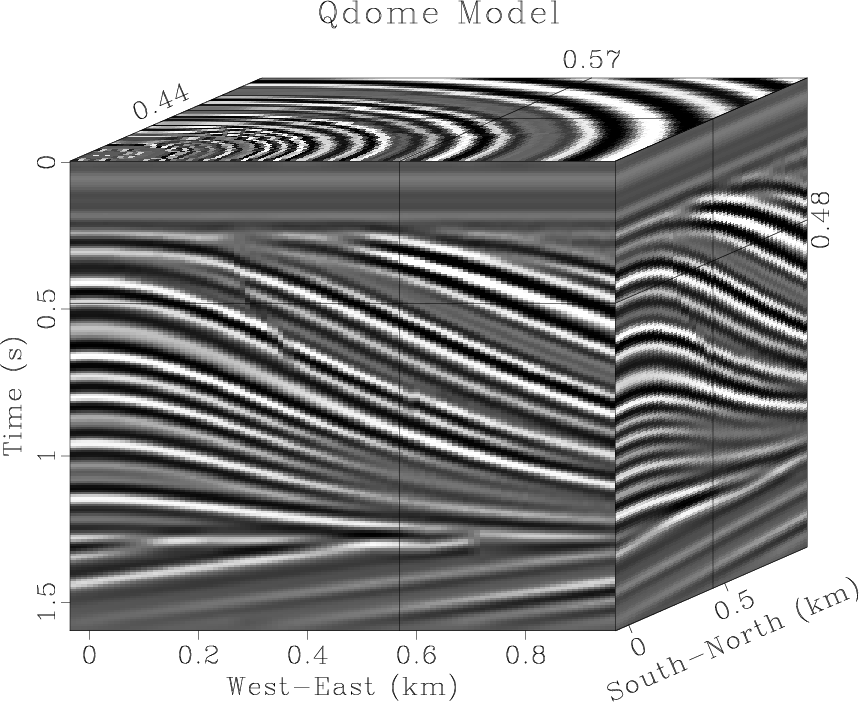sfgrey3 generates “cube” plots from 3-D data. A cube plot displays sections from a 3-D cube as faces of the cube.

Some of the options specific to this kind of display are:

• flat= The flat parameter tells sfgrey3 whether to display the cube as a flat image or in a 3-D projection. The default is flat=y. The following example from bei/fld/cube displays the same input data with both options:• frame1=, frame2=, frame3= The frame parameters select the slices from the cube to be displayed in the plot. The default is to take the first slice from the vertical and the out-of-plane axis (frame1=0 frame3=0) and the last slice from the horizontal axis (frame2=n2-1). In the following example from geo384w/hw3/gulf, the data size is n1=1000 n2=250 n3=48 and the frame parameters are frame1=500 frame2=160 frame3=10:• point1=, point2= The point1= and point2= parameters control the aspect ratio of the cube by specifying the ratio (from 0 and 1) that the front face of the cube takes from the plot in the vertical and the horizontal dimension, respectively. The default is point1=0.5 point2=0.5. The following example from sep/plane/qint has point1=0.85 point2=0.74:• movie= The movie parameter allows the user to create a movie looping over slices in a cube instead of a static picture. The value of the parameter (from 1 to 3) indicates the axis for looping or 0 (the default) for no movie. The frame increment in the movie is controled by dframe=. The following example from cwp/geo2009TTIModeSeparation/tti3 uses movie=1 to loop over the vertical axis:To generate a movie out of 4-D data, you can use sfgrey4. Here is an example: